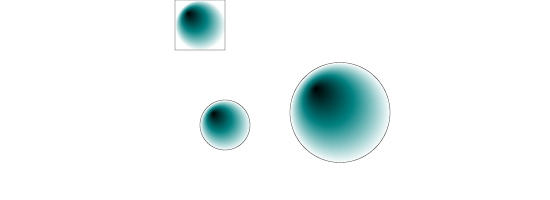# SVGBasics

Turn SVG On

## Using A Radial Gradient Fill in SVG

I find that the percentage method isolates thinking to the current gradient without concern about where it's being used. A radialGradient produces a ring (or rings) of colour instead of stripes. Instead of a line, it uses a circle whose center is (cx,cy) and radius is r. When using percentages, remember r=50% means the diameter of the circle will be 100% of the gradient.<?xml version="1.0" standalone="no"?>
<!DOCTYPE svg PUBLIC "-//W3C//DTD SVG 1.1//EN" "http://www.w3.org/Graphics/SVG/1.1/DTD/svg11.dtd">

<svg viewBox = "0 0 1100 400" version = "1.1">
<defs>
<rect id = "r1" width = "100" height = "100" stroke = "black" stroke-width = "1"/>
<circle id = "r2" cx = "100" cy = "100" r = "50" stroke = "black" stroke-width = "1"/>
<circle id = "r3" cx = "100" cy = "100" r = "100" stroke = "black" stroke-width = "1"/>
<radialGradient id = "g1" cx = "50%" cy = "50%" r = "50%">
<stop stop-color = "black" offset = "0%"/>
<stop stop-color = "teal" offset = "50%"/>
<stop stop-color = "white" offset = "100%"/>
</defs>
<use x = "350" y = "0" xlink:href = "#r1" fill = "url(#g1)"/>
<use x = "350" y = "150" xlink:href = "#r2" fill = "url(#g1)"/>
<use x = "580" y = "125" xlink:href = "#r3" fill = "url(#g1)"/>
</svg>

An interesting thing about the radial gradient is that the point from which it radiates can be moved from the center of the circle by changing the focal point (fx,fy). Here, fx=25% and fy=25%.<?xml version="1.0" standalone="no"?>
<!DOCTYPE svg PUBLIC "-//W3C//DTD SVG 1.1//EN" "http://www.w3.org/Graphics/SVG/1.1/DTD/svg11.dtd">

<svg viewBox = "0 0 1100 400" version = "1.1">
<defs>
<rect id = "r1" width = "100" height = "100" stroke = "black" stroke-width = "1"/>
<circle id = "r2" cx = "100" cy = "100" r = "50" stroke = "black" stroke-width = "1"/>
<circle id = "r3" cx = "100" cy = "100" r = "100" stroke = "black" stroke-width = "1"/>
<!-- note the 'fx' and 'fy' attributes -->
<radialGradient id = "g1" cx = "50%" cy = "50%" r = "50%" fx = "25%" fy = "25%">
<stop stop-color = "black" offset = "0%"/>
<stop stop-color = "teal" offset = "50%"/>
<stop stop-color = "white" offset = "100%"/>